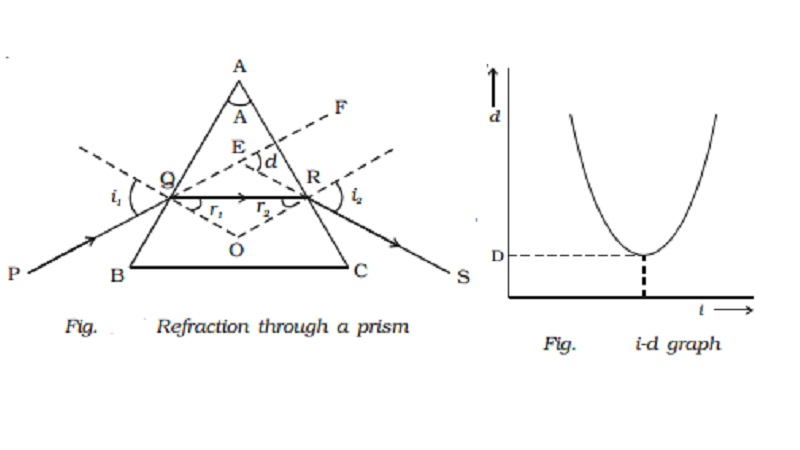Home | | Physics | | Physics | Refraction of light through a prism

# Refraction of light through a prismA prism is a transparent medium bounded by the three plane faces. Out of the three faces, one is grounded and the other two are polished. The polished faces are called refracting faces.

Prism

A prism is a transparent medium bounded by the three plane faces. Out of the three faces, one is grounded and the other two are polished. The polished faces are called refracting faces. The angle between the refracting faces is called angle of prism, or the refracting angle. The third face is called base of the prism.

Refraction of light through a prism

Fig. shows the cross section of a triangular prism ABC, placed in air. Let ?A? be the refracting angle of the prism. A ray of light PQ incident on the refracting face AB, gets refracted along QR and emerges along RS. The angle of incidence and refraction at the two faces are i1, r1 , r2  and i2  respectively. The angle between the incident ray PQ and the emergent ray RS is called angle of deviation, d. In the ∆QER, the exterior angle FER = angle  EQR + angle ERQ

d = (i1 - r1) + (i2 - r2)

d =  (i1 + i2) - (r1 + r2)           ????.(1)In the quadrilateral AQOR, the angles at Q and R are right angles

Angle Q + Angle R = 1800

A + Angle R = 1800            ????.(2)

Also, from the QOR

r1+ r2 = A ??????..(4)

Substituting in (1),

d = i1 + i2 - A

or A + d = i1 + i2 ...(5)

For a given prism and for a light of given wavelength, the angle of deviation depends upon the angle of incidence.

As the angle of incidence i gradually increases, the angle of deviation d decreases, reaches a minimum value D and then

increases. D is called the angle of minimum deviation. It will be seen from the graph (Fig. ) that there is only one angle of

incidence for which the deviation is a minimum.

At minimum deviation position the incident ray and emergent ray are symmetric with respect to the base of the prism. (i.e)the refracted ray QR is parallel to the base of the prism.

At the minimum deviation

i1 = i2  = i and r1 = r2  = r

from equation (4) 2r = A or r = A/2

and from equation (5) 2i = A + D or i = ( A+D )/2

The refractive index is ? = sin i/sin r

? = [sin((A+D) / 2)] / sin(A/2)

Study Material, Lecturing Notes, Assignment, Reference, Wiki description explanation, brief detail

Related Topics